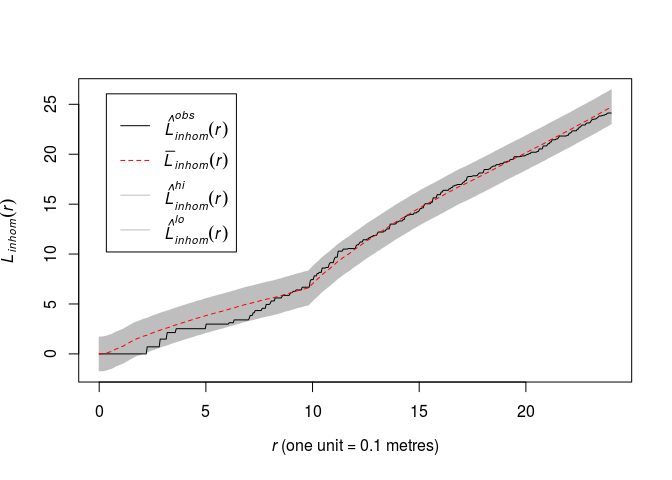# Lab 10: Gibbs processes

This session is concerned with Gibbs models for point patterns with interpoint interaction. The lecturer’s R script is available here (right click and save).

``````library(spatstat)
``````

### Exercise 1

In this question we fit a Strauss point process model to the `swedishpines` data.

1.  We need a guess at the interaction distance R. Compute and plot the L-function of the dataset and choose the value r which maximises the discrepancy L(r)−r .

We plot the above function which we want to maximize.

``````plot(Lest(swedishpines), abs(iso - r) ~ r, main = "")
``````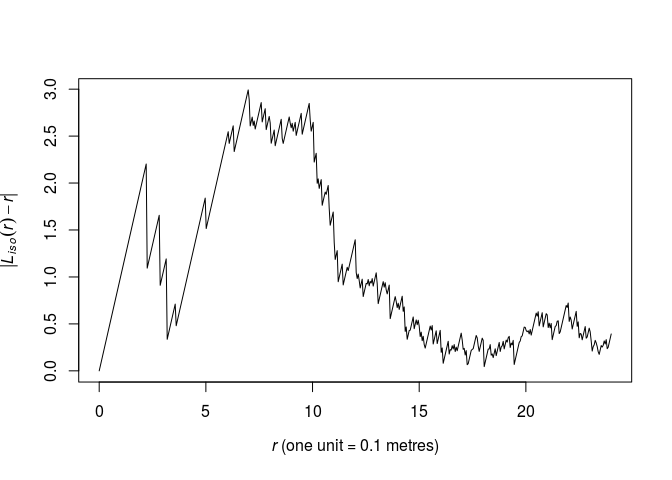As seen from the plot, the maximum lies around r = 6.5 by eye. We find the optimum explicitly like follows:

``````discrep <- function(r) {
return(abs(as.function(Lest(swedishpines))(r) - r))
}
res <- optimise(discrep, interval = c(0.1, 20), maximum = TRUE)
print(res)
``````
``````## \$maximum
##  6.984333
##
## \$objective
##  2.992058
``````
``````R <- res\$maximum
``````

This corresponds nicely with the plot.

2. Fit the stationary Strauss model with the chosen interaction distance using

``````ppm(swedishpines ~ 1, Strauss(R))
``````

where `R` is your chosen value.

3. Interpret the printout: how strong is the interaction?

4. Plot the fitted pairwise interaction function using `plot(fitin(fit))`.

As we have assigned `R`, we simply write:

``````fit <- ppm(swedishpines ~ 1, Strauss(R))
print(fit)
``````
``````## Stationary Strauss process
##
## First order term:  beta = 0.0281221
##
## Interaction distance:    6.984333
## Fitted interaction parameter gamma:   0.1434456
##
## Relevant coefficients:
## Interaction
##   -1.941799
##
## For standard errors, type coef(summary(x))
``````

As seen, the γ = 0.14 parameter is quite small. Thus there seems to be a strong negative association between points within distance R of each other. A γ of 0 implies the hard core process whereas γ = 1 implies the Poisson process and thus CSR.

The pairwise interaction function become:

``````plot(fitin(fit))
``````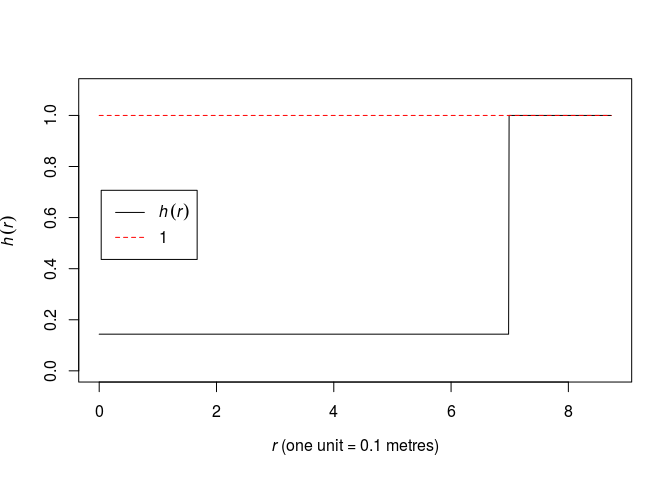### Exercise 2

In Question 1 we guesstimated the Strauss interaction distance parameter. Alternatively this parameter could be estimated by profile pseudolikelihood.

1. Look again at the plot of the L-function of `swedishpines` and determine a plausible range of possible values for the interaction distance.

``````plot(Lest(swedishpines), main = "")
``````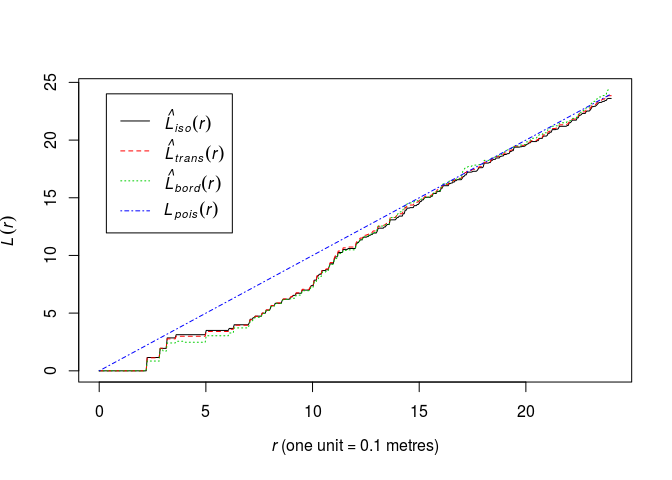A conservative range of plausible interaction distances seems to be 3 to 15 meters.

2. Generate a sequence of values equally spaced across this range, for example, if your range of plausible values was [0.05, 0.3], then type

``````rvals <- seq(0.05, 0.3, by=0.01)
``````

We generate the numbers between 3 and 12.

``````rvals <- seq(3, 12, by = 0.1)
``````
3. Construct a data frame, with one column named `r` (matching the argument name of `Strauss`), containing these values. For example

``````D <- data.frame(r = rvals)
``````

OK,

``````D <- data.frame(r = rvals)
``````
4. Execute

``````fitp <- profilepl(D, Strauss, swedishpines ~ 1)
``````

to find the maximum profile pseudolikelihood fit.

OK, let’s execute it:

``````fitp <- profilepl(D, Strauss, swedishpines ~ 1)
``````
``````## (computing rbord)

## comparing 91 models...

## 1, 2, 3, 4, 5, 6, 7, 8, 9, 10, 11, 12, 13, 14, 15, 16, 17, 18, 19, 20, 21, 22, 23, 24, 25, 26, 27, 28, 29, 30, 31, 32, 33, 34, 35, 36, 37, 38,
## 39, 40, 41, 42, 43, 44, 45, 46, 47, 48, 49, 50, 51, 52, 53, 54, 55, 56, 57, 58, 59, 60, 61, 62, 63, 64, 65, 66, 67, 68, 69, 70, 71, 72, 73, 74, 75, 76,
## 77, 78, 79, 80, 81, 82, 83, 84, 85, 86, 87, 88, 89, 90,  91.

## fitting optimal model...

## done.
``````
5. Print and plot the object `fitp`.

``````print(fitp)
``````
``````## profile log pseudolikelihood
## for model:  ppm(swedishpines ~ 1,  interaction = Strauss)
## fitted with rbord = 12
## interaction: Strauss process
## irregular parameter: r in [3, 12]
## optimum value of irregular parameter:  r = 9.8
``````
``````plot(fitp)
``````6. Compare the computed estimate of interaction distance r with your guesstimate. Compare the corresponding estimates of the Strauss interaction parameter γ.

``````(Ropt <- reach(as.ppm(fitp)))
``````
``````##  9.8
``````

The r = 9.8 is not totally inconsistent with the previous estimate of 7.

7. Extract the fitted Gibbs point process model from the object `fitp` as

``````bestfit <- as.ppm(fitp)
``````

OK, let’s do that:

``````bestfit <- as.ppm(fitp)
``````

### Exercise 3

For the Strauss model fitted in Question 1,

1. Generate and plot a simulated realisation of the fitted model using `simulate`.

``````s <- simulate(fit, drop = TRUE)
plot(s, main = "")
``````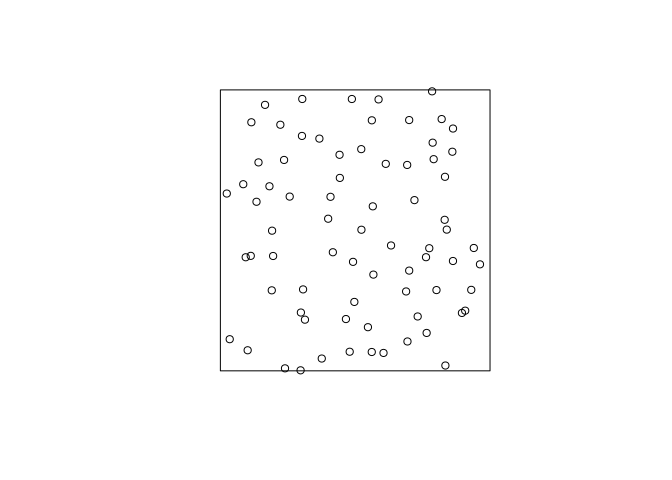2. Plot the L-function of the data along with the global simulation envelopes from 19 realisations of the fitted model.

``````plot(envelope(fit, Lest, global = TRUE, nsim = 19, nsim2 = 100), main = "")
``````
``````## Generating 119 simulated realisations of fitted Gibbs model (100 to
## estimate the mean and 19 to calculate envelopes) ...
## 1, 2, 3, 4.6.8.10.12.14.16.18.20.22.24.26.28.30.32.34.36.38.
## 40.42.44.46.48.50.52.54.56.58.60.62.64.66.68.70.72.74.76.78
## .80.82.84.86.88.90.92.94.96.98.100.102.104.106.108.110.112.114.116.
## 118 119.
##
## Done.
``````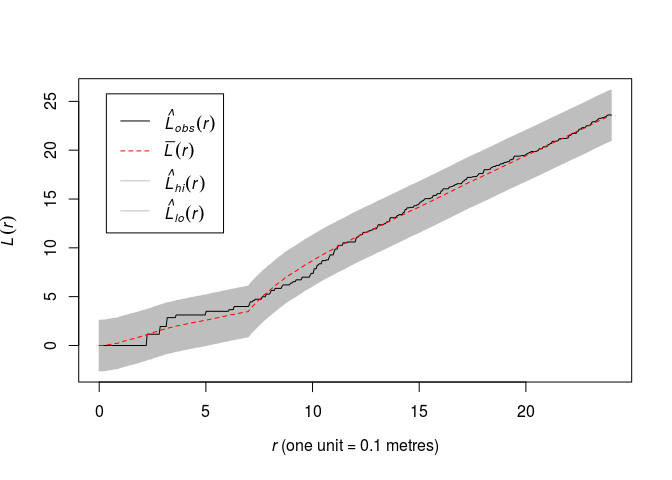### Exercise 4

1. Read the help file for `Geyer`.

See `help(Geyer)`

2. Fit a stationary Geyer saturation process to `swedishpines`, with the same interaction distance as for the Strauss model computed in Question 2, and trying different values of the saturation parameter `sat = 1, 2, 3` say.

``````ppm(swedishpines ~ 1, Geyer(r = Ropt, sat = 1))
``````
``````## Stationary Geyer saturation process
##
## First order term:  beta = 0.07472669
##
## Interaction distance:    9.8
## Saturation parameter:    1
## Fitted interaction parameter gamma:   0.1871555
##
## Relevant coefficients:
## Interaction
##   -1.675815
##
## For standard errors, type coef(summary(x))
``````
``````ppm(swedishpines ~ 1, Geyer(r = Ropt, sat = 2))
``````
``````## Stationary Geyer saturation process
##
## First order term:  beta = 0.04707047
##
## Interaction distance:    9.8
## Saturation parameter:    2
## Fitted interaction parameter gamma:   0.5242884
##
## Relevant coefficients:
## Interaction
##  -0.6457134
##
## For standard errors, type coef(summary(x))
``````
``````ppm(swedishpines ~ 1, Geyer(r = Ropt, sat = 3))
``````
``````## Stationary Geyer saturation process
##
## First order term:  beta = 0.07603509
##
## Interaction distance:    9.8
## Saturation parameter:    3
## Fitted interaction parameter gamma:   0.5261429
##
## Relevant coefficients:
## Interaction
##  -0.6421823
##
## For standard errors, type coef(summary(x))
``````
3. Fit the same model with the addition of a log-quadratic trend.

``````gfit <- ppm(swedishpines ~ polynom(x, y, 2), Geyer(r = Ropt, sat = 3))
``````
4. Plot the fitted trend and conditional intensity.

Here we use the log scale to be able to see the discs in the conditional intensity.

``````par(mfrow=c(1,2))
plot(gfit, log = TRUE, pause = FALSE)
``````### Exercise 5

Modify question 1 by using the Huang-Ogata approximate maximum likelihood algorithm (`method="ho"`) instead of maximum pseudolikelihood (the default, `method="mpl"`).

``````fit.mpl <- ppm(swedishpines ~ 1, Strauss(R), method = "mpl")
fit.ho  <- ppm(swedishpines ~ 1, Strauss(R), method = "ho")
``````
``````## Simulating... 1, 2, 3, 4, 5, 6, 7, 8, 9, 10, 11, 12, 13, 14, 15, 16, 17, 18, 19, 20, 21, 22, 23, 24, 25, 26, 27, 28, 29, 30, 31, 32, 33, 34, 35, 36, 37, 38,
## 39, 40, 41, 42, 43, 44, 45, 46, 47, 48, 49, 50, 51, 52, 53, 54, 55, 56, 57, 58, 59, 60, 61, 62, 63, 64, 65, 66, 67, 68, 69, 70, 71, 72, 73, 74, 75, 76,
## 77, 78, 79, 80, 81, 82, 83, 84, 85, 86, 87, 88, 89, 90, 91, 92, 93, 94, 95, 96, 97, 98, 99,  100.
## Done.
``````
``````print(fit.ho)
``````
``````## Stationary Strauss process
##
## First order term:  beta = 0.03025998
##
## Interaction distance:    6.984333
## Fitted interaction parameter gamma:   0.1473619
##
## Relevant coefficients:
## Interaction
##   -1.914864
##
## For standard errors, type coef(summary(x))
``````
``````print(fit.mpl)
``````
``````## Stationary Strauss process
##
## First order term:  beta = 0.0281221
##
## Interaction distance:    6.984333
## Fitted interaction parameter gamma:   0.1434456
##
## Relevant coefficients:
## Interaction
##   -1.941799
##
## For standard errors, type coef(summary(x))
``````

The fits are very similar.

### Exercise 6

Repeat Question 2 for the inhomogeneous Strauss process with log-quadratic trend. The corresponding call to `profilepl` is

``````fitp <- profilepl(D, Strauss, swedishpines ~ polynom(x,y,2))
``````
``````fitp2 <- profilepl(D, Strauss, swedishpines ~ polynom(x,y,2))
``````
``````## (computing rbord)

## comparing 91 models...

## 1, 2, 3, 4, 5, 6, 7, 8, 9, 10, 11, 12, 13, 14, 15, 16, 17, 18, 19, 20, 21, 22, 23, 24, 25, 26, 27, 28, 29, 30, 31, 32, 33, 34, 35, 36, 37, 38,
## 39, 40, 41, 42, 43, 44, 45, 46, 47, 48, 49, 50, 51, 52, 53, 54, 55, 56, 57, 58, 59, 60, 61, 62, 63, 64, 65, 66, 67, 68, 69, 70, 71, 72, 73, 74, 75, 76,
## 77, 78, 79, 80, 81, 82, 83, 84, 85, 86, 87, 88, 89, 90,  91.

## fitting optimal model...

## done.
``````
``````print(fitp)
``````
``````## profile log pseudolikelihood
## for model:  ppm(swedishpines ~ 1,  interaction = Strauss)
## fitted with rbord = 12
## interaction: Strauss process
## irregular parameter: r in [3, 12]
## optimum value of irregular parameter:  r = 9.8
``````
``````print(fitp2)
``````
``````## profile log pseudolikelihood
## for model:  ppm(swedishpines ~ polynom(x,  y,  2),  interaction = Strauss)
## fitted with rbord = 12
## interaction: Strauss process
## irregular parameter: r in [3, 12]
## optimum value of irregular parameter:  r = 9.8
``````

### Exercise 7

Repeat Question 3 for the inhomogeneous Strauss process with log-quadratic trend, using the inhomogeneous L-function `Linhom` in place of the usual L-function.

``````fit2 <- as.ppm(fitp2)
plot(envelope(fit2, Linhom, global = TRUE, nsim = 19, nsim2 = 100), main = "")
``````
``````## Generating 119 simulated realisations of fitted Gibbs model (100 to
## estimate the mean and 19 to calculate envelopes) ...
## 1, 2, 3, 4.6.8.10.12.14.16.18.20.22.24.26.28.30.32.34.36.38.
## 40.42.44.46.48.50.52.54.56.58.60.62.64.66.68.70.72.74.76.78
## .80.82.84.86.88.90.92.94.96.98.100.102.104.106.108.110.112.114.116.
## 118 119.
##
## Done.
``````